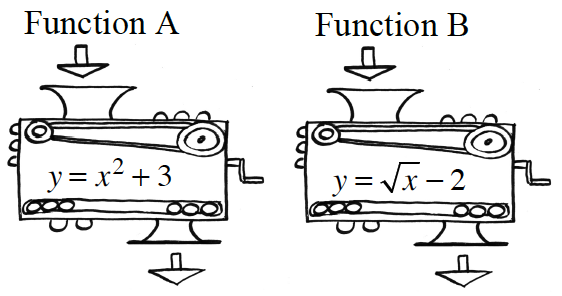Home > CCAA8 > Chapter cca1 > Lesson cca1.1.2 > Problem1-22

1-22.Function Machines Homework Help ✎

1. If an input of $-9$ is put into each of the machines at right, what is each output?

Substitute $-9$ for $x$ in each of the equations in both the machines, then solve the equations for $y$.

2. Eric wants to get an output of $0$. Can he do this with each machine? If so, how? If not, why not?

What numbers could Eric substitute in the equations to get $0$?
Is it possible to get this answer from both machines?

Function B: No solution. Do you know why?